Using Divergence theorem to find Hamaker Field (here modeled as point-wise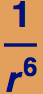) for a Finite Body

The induced dipole—dipole interaction goes as -1/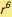, and it is this term which shows up in the Lennard-Jones potential.  This dipole-dipole interaction gives rise to the Hamaker forces between two bodies.  As a first approximation to the interaction between two bodies, one must intergrate 1/from a single point in the first body to all points in the second body.  The net interaction energy is the sum over all such points and therefore this energy produces a six-dimensional integration. There are a few cases, such a two spheres, a sphere and a seminfinite half-space, that can be worked out exactly.  However, to model the interaction energy of more complex shapes, there are no closed-form solutions and one must resort to numerical integration.

Numerical integration is a cpu-time-consuming numerical procedure—a six dimensional integration can be very expensive (time is money).  If there is a way to reduce the dimensionality of the integration, then we can reap rewards for our cleverness.  One trick is to use the divergence theorem to push the integration over a volume, to an integration over a surface. For example, we could use the divergence theorem:
∫∫∫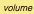∇·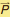dV = ∫∫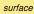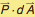We will work out such an example for a cylindrical volume interacting with a point in space, in other words, the London Interaction Potential for a finite cylinder. We will perform as many exact integrals as possible and reduce the cost of the numerical integration.  To do this, we must find a vector potentialsuch that ∇·= -1/|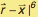whereis a position in the integrated volume and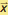is a point at which the potential is measured.  (A fairly general method to do this and similar problems can be found in Argento C; Jagota A; Carter WC ``Surface formulation for molecular interactions of macroscopic bodies'' J.Mech. Physics Solids 1997,  pp 1161-1183 .

The following is a ``guess'' at the vector potential; it will be verified as the correct one by checking its divergence.  We use (CX,CY,CZ) to represent points in the cylinder and (X,Y,Z) to be points in space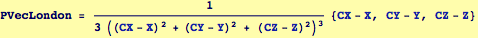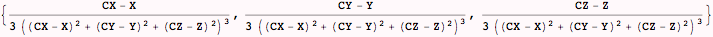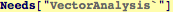The following verifies that the correct vector potential is obtained, the divergence must be taked with respect to the coodinates of the integration: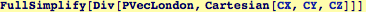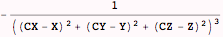We will integrate over a cylinder of radius R and length L along the z-axis, with its middle at the origin.  First, let's use the radius of the cylinder to scale all the length variables: Let (X,Y,Z)/R = (x,y,z); (CX,CY,CZ)/R = (cx,cy,cz), and L/R = λ (the cylinder's aspect ratio).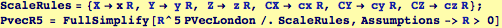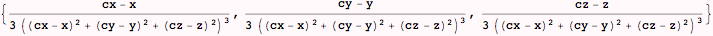Therefore, φ() =  ∫∫∫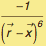dV = ∫∫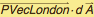= (∫∫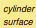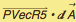+ ∫∫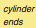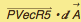)/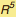is the total interaction between a point an a cylinder.  We can exploit the symmetry of the cylinder: we know that the potential will be indendent of the angular coordinate in cylindrical coordinates; it will be a function of ρ =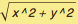and z.

We will do three integrals over the cylindrical surfaces using this expression to define the cylinder: (cx,cy,cz) = (Cos[θ], Sin[θ], cz):
The cylindrical surface  is the domain  θ ∈ (0, 2π), cz ∈ (-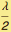,)
The two caps r ∈ (0,1),  θ ∈ (0, 2π), cz=±Created by Wolfram Mathematica 6.0  (23 October 2007)